Theory and Modern Applications

# Oscillation of impulsive functional differential equations with oscillatory potentials and Riemann-Stieltjes integrals

## Abstract

This paper addresses the oscillation problem of a class of impulsive differential equations with delays and Riemann-Stieltjes integrals that cover many equations in the literature. In the case of oscillatory potentials, both El-Sayed type and Kamenev type oscillation criteria are established by overcoming the difficulty caused by impulses and oscillatory potentials in the estimation of the delayed argument. The main results not only generalize some existing results but also drop a restrictive condition imposed on impulse constants. Finally, two examples are presented to illustrate the theoretical results.

MSC:34K11.

## 1 Introduction

Recent years have witnessed a rapid progress in the theory of impulsive differential equations which provide a natural description of the motion of several real world processes subject to short time perturbations. Due to many applications in physics, chemistry, population dynamics, ecology, biological systems, control theory, etc. , the theory of impulsive differential equations has been extensively studied in .

We are here concerned with the oscillation problem of impulsive functional differential equations. Compared to equations without impulses, the oscillation of impulsive differential equations receives less attention . In this paper, we investigate the oscillation of the following impulsive differential equation with delay and Riemann-Stieltjes integral:

$\left\{\begin{array}{c}{\left(r\left(t\right){x}^{\prime }\left(t\right)\right)}^{\prime }+q\left(t\right)x\left(t\right)+{\int }_{0}^{h}p\left(t,s\right){|x\left(\tau \left(t,s\right)\right)|}^{\alpha \left(s\right)}sgnx\left(\tau \left(t,s\right)\right)\phantom{\rule{0.2em}{0ex}}d\xi \left(s\right)=e\left(t\right),\phantom{\rule{1em}{0ex}}t\ne {t}_{k},\hfill \\ x\left({t}_{k}^{+}\right)={c}_{k}x\left({t}_{k}^{-}\right),\phantom{\rule{2em}{0ex}}{x}^{\prime }\left({t}_{k}^{+}\right)={d}_{k}{x}^{\prime }\left({t}_{k}^{-}\right),\hfill \end{array}$
(1)

where $t\ge {t}_{0}$, $0; ${\int }_{0}^{h}f\left(s\right)\phantom{\rule{0.2em}{0ex}}d\xi \left(s\right)$ denotes the Riemann-Stieltjes integral of the function f on $\left[0,h\right]$ with respect to ξ, and $\xi :\left[0,h\right]\to R$ is nondecreasing; $\alpha \left(s\right)$ is a strictly increasing continuous function on $\left[0,h\right]$ satisfying $0\le \alpha \left(0\right)<1<\alpha \left(h\right)$; ${\left\{{t}_{k}\right\}}_{k=1}^{\mathrm{\infty }}$ denotes the sequence of impulse moments satisfying $0\le {t}_{0}<{t}_{1}<{t}_{2}<\cdots <{t}_{k}<\cdots$ and ${lim}_{k\to \mathrm{\infty }}{t}_{k}=+\mathrm{\infty }$; ${c}_{k}$, ${d}_{k}$ are positive impulse constants, and ${c}_{k}\ge 1$; $r\in {C}^{1}\left[{t}_{0},\mathrm{\infty }\right)$ with $r\left(t\right)>0$, $q,e\in C\left[{t}_{0},\mathrm{\infty }\right)$, and $p\in C\left(\left[{t}_{0},\mathrm{\infty }\right)×\left[0,h\right]\right)$; the time delay $\tau \left(t,s\right):\left[{t}_{0},\mathrm{\infty }\right)×\left[0,h\right]\to \left[\sigma ,\mathrm{\infty }\right)$ with $\sigma \le {t}_{0}$ is continuous, $\tau \left(t,s\right)\le t$ and ${lim}_{t\to +\mathrm{\infty }}\tau \left(t,s\right)=+\mathrm{\infty }$ for $s\in \left[0,h\right]$.

With the choice of $\xi \left(s\right)$, $\alpha \left(s\right)$ and $\tau \left(t,s\right)$, we see that Eq. (1) reduces to many particular forms considered in the literature. Throughout this paper, we denote

$x\left({t}_{k}^{±}\right)=\underset{t\to {t}_{k}±}{lim}x\left(t\right),\phantom{\rule{2em}{0ex}}{x}^{\prime }\left({t}_{k}^{±}\right)=\underset{\mathrm{\Delta }t\to 0±}{lim}\frac{x\left({t}_{k}+\mathrm{\Delta }t\right)-x\left({t}_{k}\right)}{\mathrm{\Delta }t}.$

Let $J\subset \mathbb{R}$ be an interval. Define . By a solution of Eq. (1), we mean a function $x\left(t\right)\in PC\left(\left[{t}_{0},\mathrm{\infty }\right),\mathbb{R}\right)$ such that ${x}^{\prime }\left(t\right),{x}^{″}\left(t\right)\in PC\left(\left[{t}_{0},\mathrm{\infty }\right),\mathbb{R}\right)$, and $x\left(t\right)$ satisfies Eq. (1) for $t\in \left[{t}_{0},\mathrm{\infty }\right)$. A solution of Eq. (1) is said to be oscillatory if it is defined on some ray $\left[T,\mathrm{\infty }\right)$ with $T\ge {t}_{0}$, and has arbitrarily large zeros. Otherwise, it is called non-oscillatory. Eq. (1) is said to be oscillatory if all of its nonconstant solutions defined for all large $T>0$ are oscillatory.

Recently, there have been many papers devoted to the oscillation problem for some particular cases of Eq. (1). When there are no impulses and $\tau \left(t,s\right)\equiv \tau \left(t\right)$, Sun and Kong  established some interval oscillation criteria for the following equation:

${\left(r\left(t\right){x}^{\prime }\left(t\right)\right)}^{\prime }+q\left(t\right)x\left(t\right)+{\int }_{0}^{h}p\left(t,s\right){|x\left(\tau \left(t\right)\right)|}^{\alpha \left(s\right)}sgnx\left(\tau \left(t\right)\right)\phantom{\rule{0.2em}{0ex}}d\xi \left(s\right)=e\left(t\right),$
(2)

which generalize and improve some results for second-order differential equations with mixed nonlinearities in [22, 23].

For the case of impulse, Özbekler and Zafer [11, 12] studied forced oscillation of a class of super-half-linear differential equations. Without considering the influence of forced term, some oscillation criteria were given in . Liu and Xu  studied the oscillation of a forced mixed type Emden-Fowler equation (a particular case of Eq. (2))

$\left\{\begin{array}{c}{\left(r\left(t\right){x}^{\prime }\left(t\right)\right)}^{\prime }+q\left(t\right)x\left(t\right)+{\sum }_{i=1}^{n}{p}_{i}\left(t\right){|x\left(t\right)|}^{{\alpha }_{i}-1}x\left(t\right)=e\left(t\right),\phantom{\rule{1em}{0ex}}t\ne {\tau }_{k},\hfill \\ x\left({\tau }_{k}^{+}\right)={c}_{k}x\left({\tau }_{k}^{-}\right),\phantom{\rule{2em}{0ex}}{x}^{\prime }\left({\tau }_{k}^{+}\right)={d}_{k}{x}^{\prime }\left({\tau }_{k}^{-}\right),\hfill \end{array}$
(3)

where ${\left\{{\tau }_{k}\right\}}_{k=1}^{\mathrm{\infty }}$ is the sequence of impulse moments, ${\alpha }_{1}>\cdots >{\alpha }_{m}>1>{\alpha }_{m+1}>\cdots >{\alpha }_{n}>0$, r, q, ${p}_{i}$, e are real valued continuous functions on $\left[{t}_{0},\mathrm{\infty }\right)$ and $r\left(t\right)>0$. We note that a restrictive condition is imposed on impulse constants that ${d}_{k}\ge {c}_{k}$ in . Some oscillation results for the second-order forced mixed nonlinear impulsive differential equation were also established in [18, 19].

Zafer  investigated a class of second-order sublinear delay impulses differential equation

$\left\{\begin{array}{c}{x}^{″}\left(t\right)+p\left(t\right)x\left(t\right)+p\left(t\right){|x\left(\tau \left(t\right)\right)|}^{\alpha -1}x\left(\tau \left(t\right)\right)=0,\phantom{\rule{1em}{0ex}}t\ne {\theta }_{k},\hfill \\ \mathrm{\Delta }{x}^{\prime }\left(t\right){|}_{t={\theta }_{k}}+{q}_{k}{|x\left(\tau \left({\theta }_{k}\right)\right)|}^{\alpha -1}x\left(\tau \left({\theta }_{k}\right)\right)=0,\hfill \\ \mathrm{\Delta }x\left(t\right){|}_{t={\theta }_{k}}=0,\hfill \end{array}$
(4)

where ${\left\{{\theta }_{k}\right\}}_{k=1}^{\mathrm{\infty }}$ is the sequence of impulse moments and $0<\alpha <1$. The author established oscillation criteria in two cases of $\tau \left(t\right)\le t$ and $\tau \left(t\right)=t$. We see that $x\left(t\right)$ is additionally assumed to be continuous in .

In our recent paper , we further extend the main results in  to Eq. (1) with $\tau \left(t,s\right)=t$. However, the oscillation problem of Eq. (1) remains untouched in  for the case of oscillatory potentials.

Generally speaking, some ideas to oscillation of differential equations without impulses can also be applied to impulsive differential equations. For example, the idea to interval oscillation in  and the idea of dealing with mixed nonlinearities in . However, when the potentials q, p and e are allowed to change signs, it is difficult to deal with the delayed argument $x\left(\tau \left(t,s\right)\right)$ for Eq. (1) as that for differential equations without impulses in . In this paper, we will overcome difficulties caused by oscillatory potentials, delayed argument and impulses, and establish both El-Sayed type and Kamenev type interval oscillation criteria for Eq. (1).

The main contribution of this paper is threefold. First, in the case of oscillatory potentials, we present an estimation on $x\left(\tau \left(t,s\right)\right)/x\left(t\right)$ in a bounded interval, which plays a key role in the proof of the main results. Second, the redundant restriction on impulse constants ${c}_{k}$ and ${d}_{k}$ that ${d}_{k}\ge {c}_{k}$ is removed by introducing particular El-Sayed type functions in  and using Kong’s technique in  many times based on the number of impulse moments in a bounded interval. Finally, both impulse, delay and Riemann-Stieltjes integral are taken into consideration in this paper. Therefore, most of mixed type Emden-Fowler equations considered in the literature are included as special cases.

The remainder of this paper is organized as follows. In Section 2, some important lemmas are given. Interval oscillation criteria of the El-Sayed type and the Kong type are established in Section 3. Finally, two examples are given in Section 4.

## 2 Preliminaries

Throughout this paper, we suppose that there are limited impulse moments in any bounded time interval. For the sake of convenience, we introduce the following notations. Denote

$\phi \left(a\right)=min\left\{k|{t}_{k}>a,{t}_{k}>{t}_{0}\right\},\phantom{\rule{2em}{0ex}}\psi \left(b\right)=max\left\{k|{t}_{0}<{t}_{k}

where $b>a>{t}_{0}$ are constants. It is easy to see that ${t}_{\phi \left(a\right)},{t}_{\phi \left(a\right)+1},{t}_{\phi \left(a\right)+2},\dots ,{t}_{\psi \left(b\right)}$ are all impulse moments in the interval $\left(a,b\right)$.

The following two lemmas are crucial in the proof of our main results.

Lemma 2.1 For given constants $b>a\ge {t}_{0}$, assume that $x\left(t\right),{x}^{\prime }\left(t\right),{x}^{″}\left(t\right)\in PC\left(\left(a,b\right),\mathbb{R}\right)$, $x\left(t\right)>0$ (<0) and ${\left(r\left(t\right){x}^{\prime }\left(t\right)\right)}^{\prime }\le 0$ (≥0) for $t\in \left(a,b\right)$. Then we have

$\frac{{x}^{\prime }\left(t\right)}{x\left(t\right)}\le \frac{1}{r\left(t\right)R\left(a,t\right)},\phantom{\rule{1em}{0ex}}t\in \left(a,b\right),$
(5)

where

$R\left(a,t\right)=\left[{\int }_{{t}_{\psi \left(t\right)}}^{t}\frac{ds}{r\left(s\right)}+\frac{1}{{d}_{\psi \left(t\right)}}{\int }_{{t}_{\psi \left(t\right)-1}}^{{t}_{\psi \left(t\right)}}\frac{ds}{r\left(s\right)}+\cdots +\left(\prod _{k=\phi \left(a\right)}^{\psi \left(t\right)}\frac{1}{{d}_{k}}\right){\int }_{a}^{{t}_{\phi \left(a\right)}}\frac{ds}{r\left(s\right)}\right].$

Proof It is sufficient to prove one of the cases when $x\left(t\right)>0$ on $\left(a,b\right)$ and ${\left(r\left(t\right){x}^{\prime }\left(t\right)\right)}^{\prime }\le 0$ for $t\in \left(a,b\right)$. The other case can be proved similarly. By Eq. (1) and ${c}_{k}\ge 1$, we have that for $t\in \left(a,b\right)$,

$\begin{array}{rcl}x\left(t\right)& \ge & x\left(t\right)-x\left({t}_{\psi \left(t\right)}^{+}\right)+x\left({t}_{\psi \left(t\right)}^{+}\right)-x\left({t}_{\psi \left(t\right)}^{-}\right)+x\left({t}_{\psi \left(t\right)}^{-}\right)\\ -x\left({t}_{\psi \left(t\right)-1}^{+}\right)+\cdots +x\left({t}_{\phi \left(a\right)}^{-}\right)-x\left(a\right)\\ =& {\int }_{{t}_{\psi \left(t\right)}}^{t}{x}^{\prime }\left(s\right)\phantom{\rule{0.2em}{0ex}}ds+\left({c}_{\psi \left(t\right)}-1\right)x\left({t}_{\psi \left(t\right)}\right)+{\int }_{{t}_{\psi \left(t\right)-1}}^{{t}_{\psi \left(t\right)}}{x}^{\prime }\left(s\right)\phantom{\rule{0.2em}{0ex}}ds+\left({c}_{\psi \left(t\right)-1}-1\right)x\left({t}_{\psi \left(t\right)-1}\right)\\ +\cdots +{\int }_{{t}_{\phi \left(a\right)}}^{{t}_{\phi \left(a\right)+1}}{x}^{\prime }\left(s\right)\phantom{\rule{0.2em}{0ex}}ds+\left({c}_{\phi \left(a\right)}-1\right)x\left({t}_{\phi \left(a\right)}\right)+{\int }_{a}^{{t}_{\phi \left(a\right)}}{x}^{\prime }\left(s\right)\phantom{\rule{0.2em}{0ex}}ds\\ \ge & {\int }_{{t}_{\psi \left(t\right)}}^{t}{x}^{\prime }\left(s\right)\phantom{\rule{0.2em}{0ex}}ds+{\int }_{{t}_{\psi \left(t\right)-1}}^{{t}_{\psi \left(t\right)}}{x}^{\prime }\left(s\right)\phantom{\rule{0.2em}{0ex}}ds+\cdots +{\int }_{{t}_{\phi \left(a\right)}}^{{t}_{\phi \left(a\right)+1}}{x}^{\prime }\left(s\right)\phantom{\rule{0.2em}{0ex}}ds+{\int }_{a}^{{t}_{\phi \left(a\right)}}{x}^{\prime }\left(s\right)\phantom{\rule{0.2em}{0ex}}ds.\end{array}$
(6)

Since ${\left(r\left(t\right){x}^{\prime }\left(t\right)\right)}^{\prime }\le 0$ for $t\in \left(a,b\right)$ and $t\ne {t}_{k}$, and ${x}^{\prime }\left({t}_{k}\right)={x}^{\prime }\left({t}_{k}^{-}\right)=\frac{1}{{d}_{k}}{x}^{\prime }\left({t}_{k}^{+}\right)$, we get from (6) that

$\begin{array}{rcl}x\left(t\right)& \ge & {\int }_{{t}_{\psi \left(t\right)}}^{t}\frac{1}{r\left(s\right)}r\left(s\right){x}^{\prime }\left(s\right)\phantom{\rule{0.2em}{0ex}}ds+{\int }_{{t}_{\psi \left(t\right)-1}}^{{t}_{\psi \left(t\right)}}\frac{1}{r\left(s\right)}r\left(s\right){x}^{\prime }\left(s\right)\phantom{\rule{0.2em}{0ex}}ds+\cdots +{\int }_{a}^{{t}_{\phi \left(a\right)}}\frac{1}{r\left(s\right)}r\left(s\right){x}^{\prime }\left(s\right)\phantom{\rule{0.2em}{0ex}}ds\\ \ge & r\left(t\right){x}^{\prime }\left(t\right){\int }_{{t}_{\psi \left(t\right)}}^{t}\frac{1}{r\left(s\right)}\phantom{\rule{0.2em}{0ex}}ds+r\left({t}_{\psi \left(t\right)}\right){x}^{\prime }\left({t}_{\psi \left(t\right)}^{-}\right){\int }_{{t}_{\psi \left(t\right)-1}}^{{t}_{\psi \left(t\right)}}\frac{1}{r\left(s\right)}\phantom{\rule{0.2em}{0ex}}ds\\ +\cdots +r\left({t}_{\phi \left(a\right)}\right){x}^{\prime }\left({t}_{\phi \left(a\right)}^{-}\right){\int }_{a}^{{t}_{\phi \left(a\right)}}\frac{1}{r\left(s\right)}\phantom{\rule{0.2em}{0ex}}ds\\ \ge & r\left(t\right){x}^{\prime }\left(t\right){\int }_{{t}_{\psi \left(t\right)}}^{t}\frac{1}{r\left(s\right)}\phantom{\rule{0.2em}{0ex}}ds+\frac{r\left(t\right){x}^{\prime }\left(t\right)}{{d}_{\psi \left(t\right)}}{\int }_{{t}_{\psi \left(t\right)-1}}^{{t}_{\psi \left(t\right)}}\frac{1}{r\left(s\right)}\phantom{\rule{0.2em}{0ex}}ds\\ +\cdots +\frac{r\left(t\right){x}^{\prime }\left(t\right)}{{d}_{\psi \left(t\right)}{d}_{\psi \left(t\right)-1}\cdots {d}_{\phi \left(a\right)}}{\int }_{a}^{{t}_{\phi \left(a\right)}}\frac{1}{r\left(s\right)}\phantom{\rule{0.2em}{0ex}}ds\\ =& r\left(t\right){x}^{\prime }\left(t\right)R\left(a,t\right),\end{array}$

i.e.,

$\frac{{x}^{\prime }\left(t\right)}{x\left(t\right)}\le \frac{1}{r\left(t\right)R\left(a,t\right)},\phantom{\rule{1em}{0ex}}t\in \left(a,b\right).$

This completes the proof of Lemma 2.1. □

Remark 1 We see that $R\left(a,t\right)$ is a piecewise continuous function on $\left(a,b\right)$. When there is no impulse moment on $\left(a,t\right)$, $R\left(a,t\right)={\int }_{a}^{t}\frac{1}{r\left(s\right)}\phantom{\rule{0.2em}{0ex}}ds$. When there is only one impulse moment ${t}_{k}$ on $\left(a,b\right)$, we have

$R\left(a,t\right)=\left\{\begin{array}{cc}{\int }_{a}^{t}\frac{ds}{r\left(s\right)},\hfill & t\in \left(a,{t}_{k}\right],\hfill \\ {\int }_{{t}_{k}}^{t}\frac{ds}{r\left(s\right)}+\frac{1}{{d}_{k}}{\int }_{a}^{{t}_{k}}\frac{ds}{r\left(s\right)},\hfill & t\in \left({t}_{k},b\right).\hfill \end{array}$

Lemma 2.2 For given constants $b>a\ge {t}_{0}$, assume that $x\left(t\right),{x}^{\prime }\left(t\right),{x}^{″}\left(t\right)\in PC\left(\left(a,b\right),\mathbb{R}\right)$, $x\left(t\right)>0$ (<0) and ${\left(r\left(t\right){x}^{\prime }\left(t\right)\right)}^{\prime }\le 0$ (≥0) for $t\in \left({\tau }_{ab},b\right)$, where ${\tau }_{ab}=min\left\{\tau \left(t,s\right):\left(t,s\right)\in \left(a,b\right)×\left[0,h\right]\right\}$. Then we have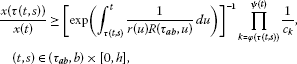(7)

where $R\left({\tau }_{ab},t\right)$ is defined as in Lemma  2.1.

Proof It is sufficient to prove the case when $x\left(t\right)>0$ and ${\left(r\left(t\right){x}^{\prime }\left(t\right)\right)}^{\prime }\le 0$ for $t\in \left({\tau }_{ab},b\right)$. By Lemma 2.1, we obtain

$\frac{{x}^{\prime }\left(t\right)}{x\left(t\right)}\le \frac{1}{r\left(t\right)R\left({\tau }_{ab},t\right)},\phantom{\rule{1em}{0ex}}t\in \left({\tau }_{ab},b\right).$
(8)

Noting that

$lnx\left({t}_{k}^{+}\right)-lnx\left({t}_{k}^{-}\right)=ln\frac{x\left({t}_{k}^{+}\right)}{x\left({t}_{k}^{-}\right)}=ln{c}_{k},$

and ${t}_{\phi \left(\tau \left(t,s\right)\right)},{t}_{\phi \left(\tau \left(t,s\right)\right)+1},\dots ,{t}_{\psi \left(t\right)}\in \left(\tau \left(t,s\right),t\right)$ are all impulse moments, we have that

$\begin{array}{rcl}ln\frac{x\left(t\right)}{x\left(\tau \left(t,s\right)\right)}& =& \left(lnx\left(t\right)-lnx\left({t}_{\psi \left(t\right)}^{+}\right)\right)+\left(lnx\left({t}_{\psi \left(t\right)}^{+}\right)-lnx\left({t}_{\psi \left(t\right)}^{-}\right)\right)\\ +\left(lnx\left({t}_{\psi \left(t\right)}^{-}\right)-lnx\left({t}_{\psi \left(t\right)-1}^{+}\right)\right)+\cdots +\left(lnx\left({t}_{\phi \left(\tau \left(t,s\right)\right)}^{+}\right)-lnx\left({t}_{\phi \left(\tau \left(t,s\right)\right)}^{-}\right)\right)\\ +\left(lnx\left({t}_{\phi \left(\tau \left(t,s\right)\right)}^{-}\right)-lnx\left(\tau \left(t,s\right)\right)\right)\\ =& {\int }_{{t}_{\psi \left(t\right)}}^{t}\frac{{x}^{\prime }\left(s\right)}{x\left(s\right)}\phantom{\rule{0.2em}{0ex}}ds+{\int }_{{t}_{\psi \left(t\right)-1}}^{{t}_{\psi \left(t\right)}}\frac{{x}^{\prime }\left(s\right)}{x\left(s\right)}\phantom{\rule{0.2em}{0ex}}ds+\cdots +{\int }_{\tau \left(t,s\right)}^{{t}_{\phi \left(\tau \left(t,s\right)\right)}}\frac{{x}^{\prime }\left(s\right)}{x\left(s\right)}\phantom{\rule{0.2em}{0ex}}ds+\sum _{k=\phi \left(\tau \left(t,s\right)\right)}^{\psi \left(t\right)}ln{c}_{k},\end{array}$

i.e.,

$ln\frac{x\left(t\right)}{x\left(\tau \left(t,s\right)\right)}={\int }_{\tau \left(t,s\right)}^{t}\frac{{x}^{\prime }\left(s\right)}{x\left(s\right)}\phantom{\rule{0.2em}{0ex}}ds+\sum _{k=\phi \left(\tau \left(t,s\right)\right)}^{\psi \left(t\right)}ln{c}_{k}.$

Integrating (8) from $\tau \left(t,s\right)$ to t with $\left(t,s\right)\in \left({\tau }_{ab},b\right)×\left[0,h\right]$, we get

$ln\frac{x\left(t\right)}{x\left(\tau \left(t,s\right)\right)}\le {\int }_{\tau \left(t,s\right)}^{t}\frac{1}{r\left(u\right)R\left({\tau }_{ab},u\right)}\phantom{\rule{0.2em}{0ex}}du+\sum _{k=\phi \left(\tau \left(t,s\right)\right)}^{\psi \left(t\right)}ln{c}_{k}.$

Therefore,

$\frac{x\left(t\right)}{x\left(\tau \left(t,s\right)\right)}\le exp\left({\int }_{\tau \left(t,s\right)}^{t}\frac{1}{r\left(u\right)R\left({\tau }_{ab},u\right)}\phantom{\rule{0.2em}{0ex}}du\right)\prod _{k=\phi \left(\tau \left(t,s\right)\right)}^{\psi \left(t\right)}{c}_{k},$

which implies (7). The proof of Lemma 2.2 is complete. □

Remark 2 For the sake of convenience, we denote

$m\left(t,s;a,b\right)\triangleq {\left[exp\left({\int }_{\tau \left(t,s;a,b\right)}^{t}\frac{1}{r\left(u\right)R\left({\tau }_{ab},u\right)}\phantom{\rule{0.2em}{0ex}}du\right)\right]}^{-1}\prod _{k=\phi \left(\tau \left(t,s\right)\right)}^{\psi \left(t\right)}\frac{1}{{c}_{k}}.$
(9)

It is easy to see that $m\left(t,s;a,b\right)$ is a piecewise continuous function on $\left[a,b\right]$ for given $s\in \left[0,h\right]$.

We denote by ${L}_{\xi }\left(0,h\right)$ the set of Riemann-Stieltjes integrable functions on $\left[0,h\right]$ with respect to ξ. Let $c\in \left(0,h\right)$ such that $\alpha \left(c\right)=1$. We further assume that ${\alpha }^{-1}\in {L}_{\xi }\left(0,h\right)$ such that

${\int }_{0}^{c}d\xi \left(s\right)>0,\phantom{\rule{2em}{0ex}}{\int }_{c}^{h}d\xi \left(s\right)>0.$

We see that the condition ${\alpha }^{-1}\in {L}_{\xi }\left(0,h\right)$ is satisfied if either $\alpha \left(0\right)>0$ or $\alpha \left(s\right)\to 0$ ‘slowly’ as $s\to 0+$, or $\xi \left(s\right)$ is constant in a right neighborhood of 0.

The following two lemmas are given in .

Lemma 2.3 Let

${l}_{1}=\left({\int }_{c}^{h}{\alpha }^{-1}\left(s\right)\phantom{\rule{0.2em}{0ex}}d\xi \left(s\right)\right){\left({\int }_{c}^{h}d\xi \left(s\right)\right)}^{-1},$

and

${l}_{2}=\left({\int }_{0}^{c}{\alpha }^{-1}\left(s\right)\phantom{\rule{0.2em}{0ex}}d\xi \left(s\right)\right){\left({\int }_{0}^{c}d\xi \left(s\right)\right)}^{-1}.$

Then for any $\delta \in \left({l}_{1},{l}_{2}\right)$, there exists $\eta \in {L}_{\xi }\left(0,b\right)$ such that $\eta \left(s\right)>0$ on $\left[0,h\right]$,

${\int }_{0}^{h}\alpha \left(s\right)\eta \left(s\right)\phantom{\rule{0.2em}{0ex}}d\xi \left(s\right)=1,$
(10)

and

${\int }_{0}^{h}\eta \left(s\right)\phantom{\rule{0.2em}{0ex}}d\xi \left(s\right)=\delta .$
(11)

Lemma 2.4 Let $\upsilon \in C\left[0,h\right]$ and $\eta \in {L}_{\xi }\left(0,h\right)$ satisfying $\upsilon \ge 0$, $\eta >0$ on $\left[0,h\right]$ and ${\int }_{0}^{h}\eta \left(s\right)\phantom{\rule{0.2em}{0ex}}d\xi \left(s\right)=1$. Then

${\int }_{0}^{h}\eta \left(s\right)\upsilon \left(s\right)\phantom{\rule{0.2em}{0ex}}d\xi \left(s\right)\ge exp\left({\int }_{0}^{h}\eta \left(s\right)ln\left[\upsilon \left(s\right)\right]\phantom{\rule{0.2em}{0ex}}d\xi \left(s\right)\right),$
(12)

where we use the convention that $ln0=-\mathrm{\infty }$ and ${e}^{-\mathrm{\infty }}=0$.

## 3 Main results

Let

$\begin{array}{rcl}\mathrm{\Omega }\left({a}_{j},{b}_{j}\right)& =& \left\{\omega \in {C}^{1}\left[{a}_{j},{b}_{j}\right],\omega \left(t\right)\not\equiv 0,\\ \omega \left({a}_{j}\right)=\omega \left({t}_{\phi \left({a}_{j}\right)}\right)=\cdots =\omega \left({t}_{\psi \left({b}_{j}\right)}\right)=\omega \left({b}_{j}\right)=0\right\},\end{array}$

for $j=1,2$.

Theorem 3.1 If for any $T\ge {t}_{0}$, there exist constants ${a}_{j},{b}_{j}\ge {t}_{0}$ for $j=1,2$ such that $T\le {a}_{1}<{b}_{1}\le {a}_{2}<{b}_{2}$, and

$\left\{\begin{array}{c}p\left(t,s\right)\ge 0,\phantom{\rule{1em}{0ex}}\left(t,s\right)\in \left({a}_{1},{b}_{1}\right)×\left[0,h\right]\cup \left({a}_{2},{b}_{2}\right)×\left[0,h\right];\hfill \\ e\left(t\right)\le 0,\phantom{\rule{1em}{0ex}}t\in \left({a}_{1},{b}_{1}\right);\phantom{\rule{2em}{0ex}}e\left(t\right)\ge 0,\phantom{\rule{1em}{0ex}}t\in \left({a}_{2},{b}_{2}\right).\hfill \end{array}$

For each $\delta \in \left({l}_{1},{l}_{2}\right)$, let $\eta \in {L}_{\xi }\left(0,h\right)$ be defined as in Lemma  2.3. Assume further that there exist $\omega \in \mathrm{\Omega }\left({a}_{j},{b}_{j}\right)$ and $\rho \in {C}^{1}\left(\left({a}_{1},{b}_{1}\right)\cup \left({a}_{2},{b}_{2}\right),\left(0,\mathrm{\infty }\right)\right)$ such that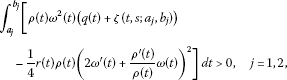where

$\zeta \left(t,s;{a}_{j},{b}_{j}\right)={\left[\frac{|e\left(t\right)|}{1-\delta }\right]}^{1-\delta }exp\left({\int }_{0}^{h}\eta \left(s\right)ln\frac{p\left(t,s\right){\left[m\left(t,s;{a}_{j},{b}_{j}\right)\right]}^{\alpha \left(s\right)}}{\eta \left(s\right)}\phantom{\rule{0.2em}{0ex}}d\xi \left(s\right)\right),$
(13)

and $m\left(t,s;{a}_{j},{b}_{j}\right)$ is defined as (9). Then Eq. (1) is oscillatory.

Proof Assume, for the sake of contradiction, that there exists a solution $x\left(t\right)$ of Eq. (1) which does not have zero in $\left({a}_{1},{b}_{1}\right)\cup \left({a}_{2},{b}_{2}\right)$. Without loss of generality, we may assume that $x\left(t\right)>0$ for $t\in \left({a}_{1},{b}_{1}\right)$. When $x\left(t\right)<0$ for $t\in \left({a}_{2},{b}_{2}\right)$, the proof follows the same argument by using the interval $\left({a}_{2},{b}_{2}\right)$ instead of $\left({a}_{1},{b}_{1}\right)$. Put

$u\left(t\right)=-\rho \left(t\right)\frac{r\left(t\right){x}^{\prime }\left(t\right)}{x\left(t\right)},\phantom{\rule{1em}{0ex}}t\in \left({a}_{1},{b}_{1}\right).$
(14)

We have

$\begin{array}{rcl}{u}^{\prime }\left(t\right)& =& -{\rho }^{\prime }\left(t\right)\frac{r\left(t\right){x}^{\prime }\left(t\right)}{x\left(t\right)}-\rho \left(t\right)\frac{{\left(r\left(t\right){x}^{\prime }\left(t\right)\right)}^{\prime }}{x\left(t\right)}+\rho \left(t\right)\frac{r\left(t\right){\left({x}^{\prime }\left(t\right)\right)}^{2}}{{x}^{2}\left(t\right)}\\ =& \frac{{\rho }^{\prime }\left(t\right)}{\rho \left(t\right)}u\left(t\right)+\rho \left(t\right)q\left(t\right)+\frac{\rho \left(t\right)}{x\left(t\right)}\left({\int }_{0}^{h}p\left(t,s\right){\left[x\left(\tau \left(t,s\right)\right)\right]}^{\alpha \left(s\right)}\phantom{\rule{0.2em}{0ex}}d\xi \left(s\right)-e\left(t\right)\right)\\ +\frac{{u}^{2}\left(t\right)}{\rho \left(t\right)r\left(t\right)}.\end{array}$
(15)

By Lemma 2.2, we have that for $t\in \left({a}_{1},{b}_{1}\right)$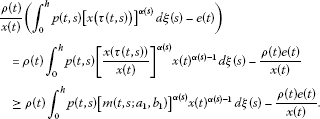Similar to the analysis in the proof of Theorem 2.1 in , we can get from Lemmas 2.3 and 2.4 that

${u}^{\prime }\left(t\right)\ge \frac{{\rho }^{\prime }\left(t\right)}{\rho \left(t\right)}u\left(t\right)+\rho \left(t\right)q\left(t\right)+\rho \left(t\right)\zeta \left(t,s;{a}_{1},{b}_{1}\right)+\frac{{u}^{2}\left(t\right)}{\rho \left(t\right)r\left(t\right)},\phantom{\rule{1em}{0ex}}t\in \left({a}_{1},{b}_{1}\right),t\ne {t}_{k}.$
(16)

By the definition of $\omega \left(t\right)$, multiplying both sides of (16) by ${\omega }^{2}\left(t\right)$, integrating over $\left({a}_{1},{b}_{1}\right)$ and using integration by parts, we get

$\begin{array}{rcl}0& \ge & {\int }_{{a}_{1}}^{{b}_{1}}\rho \left(t\right){\omega }^{2}\left(t\right)\left(q\left(t\right)+\zeta \left(t,s;{a}_{1},{b}_{1}\right)\right)-\frac{1}{4}r\left(t\right)\rho \left(t\right){\left(2{\omega }^{\prime }\left(t\right)+\frac{{\rho }^{\prime }\left(t\right)}{\rho \left(t\right)}\omega \left(t\right)\right)}^{2}\phantom{\rule{0.2em}{0ex}}dt\\ +{\int }_{{a}_{1}}^{{b}_{1}}\frac{1}{r\left(t\right)\rho \left(t\right)}{\left[u\left(t\right)\omega \left(t\right)+\frac{1}{2}r\left(t\right)\rho \left(t\right)\left(2{\omega }^{\prime }\left(t\right)+\frac{{\rho }^{\prime }\left(t\right)}{\rho \left(t\right)}\omega \left(t\right)\right)\right]}^{2}\phantom{\rule{0.2em}{0ex}}dt.\end{array}$
(17)

Noting that the second term of the right-hand side of (17) is nonnegative, we get

$0\ge {\int }_{{a}_{1}}^{{b}_{1}}\left[\rho \left(t\right){\omega }^{2}\left(t\right)\left(q\left(t\right)+\zeta \left(t,s;{a}_{1},{b}_{1}\right)\right)-\frac{1}{4}r\left(t\right)\rho \left(t\right){\left(2{\omega }^{\prime }\left(t\right)+\frac{{\rho }^{\prime }\left(t\right)}{\rho \left(t\right)}\omega \left(t\right)\right)}^{2}\right]\phantom{\rule{0.2em}{0ex}}dt.$

This contradicts the assumption. □

Next, we will establish a Kamenev type interval oscillation criterion for Eq. (1). First, we introduce a class of functions which will be used in the sequel. Denote $D=\left\{\left(t,{t}^{\ast }\right)|{t}_{0}\le {t}^{\ast }\le t\right\}$ and $H\in C\left(D,\mathbb{R}\right)$. A function H is said to belong to the class if there exist ${h}_{1},{h}_{2}\in {L}_{loc}\left(D,\mathbb{R}\right)$ satisfying the following conditions:

(A1) $H\left(t,t\right)=0$, $H\left(t,{t}^{\ast }\right)>0$ for $t>{t}^{\ast }$;

(A2) $\frac{\partial }{\partial t}H\left(t,{t}^{\ast }\right)={h}_{1}\left(t,{t}^{\ast }\right)H\left(t,{t}^{\ast }\right)$, $\frac{\partial }{\partial {t}^{\ast }}H\left(t,{t}^{\ast }\right)={h}_{2}\left(t,{t}^{\ast }\right)H\left(t,{t}^{\ast }\right)$.

For two constants θ, λ ($\theta <\lambda$), we define two operators ${P}_{1}$, ${P}_{2}$ by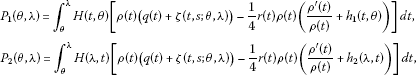where $\zeta \left(t,s;\theta ,\lambda \right)$ is defined as in Theorem 3.1.

Noticing that ${t}_{\phi \left({a}_{j}\right)},{t}_{\phi \left({a}_{j}\right)+1},{t}_{\phi \left({a}_{j}\right)+2},\dots ,{t}_{\psi \left({b}_{j}\right)}$ are all impulse moments in the interval $\left({a}_{j},{b}_{j}\right)$ for $j=1,2$, we denote the number of impulse moments between ${a}_{j}$ and ${b}_{j}$ by ${n}_{j}:={n}_{j}\left({a}_{j},{b}_{j}\right)=\psi \left({b}_{j}\right)-\phi \left({a}_{j}\right)+1$ for $j=1,2$. We also mean ${\sum }_{n=l}^{m}=0$ if $m.

Theorem 3.2 Suppose that for any $T\ge 0$, there exist nontrivial subintervals $\left({a}_{1},{b}_{1}\right)$ and $\left({a}_{2},{b}_{2}\right)$ of $\left[T,\mathrm{\infty }\right)$, satisfying the conditions of Theorem  3.1. Further assume that for $j=1,2$, there exist constants ${\chi }_{j}\in \left(\psi \left({b}_{j}\right),{b}_{j}\right)$ and a function $H\in \mathcal{H}$ such that

1. (i)

when ${n}_{j}=\psi \left({b}_{j}\right)-\phi \left({a}_{j}\right)+1$ ($j=1,2$) is an odd number,

$\begin{array}{rcl}0& <& \left\{\frac{1}{H\left({t}_{\phi \left({a}_{j}\right)},{a}_{j}\right)}{P}_{1}\left({a}_{j},{t}_{\phi \left({a}_{j}\right)}\right)\\ +\sum _{n=1}^{n=\frac{{n}_{j}-1}{2}}\frac{1}{H\left({t}_{\phi \left({a}_{j}\right)+2n},{t}_{\phi \left({a}_{j}\right)+2n-1}\right)}{P}_{1}\left({t}_{\phi \left({a}_{j}\right)+2n-1},{t}_{\phi \left({a}_{j}\right)+2n}\right)\\ +\sum _{n=0}^{n=\frac{{n}_{j}-3}{2}}\frac{{c}_{\phi \left({a}_{j}\right)+2n}}{{d}_{\phi \left({a}_{j}\right)+2n}}\frac{1}{H\left({t}_{\phi \left({a}_{j}\right)+2n+1},{t}_{\phi \left({a}_{j}\right)+2n}\right)}{P}_{2}\left({t}_{\phi \left({a}_{j}\right)+2n},{t}_{\phi \left({a}_{j}\right)+2n+1}\right)\\ +\frac{{c}_{\phi \left({a}_{j}\right)+{n}_{j}-1}}{{d}_{\phi \left({a}_{j}\right)+{n}_{j}-1}}\frac{1}{H\left({b}_{j},{t}_{\phi \left({a}_{j}\right)+{n}_{j}-1}\right)}{P}_{2}\left({t}_{\phi \left({a}_{j}\right)+{n}_{j}-1},{b}_{j}\right)\right\};\end{array}$
(18)
2. (ii)

when ${n}_{j}$ ($j=1,2$) is an even number,

$\begin{array}{rcl}0& <& \left\{\frac{1}{H\left({t}_{\phi \left({a}_{j}\right)},{a}_{j}\right)}{P}_{1}\left({a}_{j},{t}_{\phi \left({a}_{j}\right)}\right)\\ +\sum _{n=1}^{n=\frac{{n}_{j}-2}{2}}\frac{1}{H\left({t}_{\phi \left({a}_{j}\right)+2n},{t}_{\phi \left({a}_{j}\right)+2n-1}\right)}{P}_{1}\left({t}_{\phi \left({a}_{j}\right)+2n-1},{t}_{\phi \left({a}_{j}\right)+2n}\right)\\ +\sum _{n=0}^{n=\frac{{n}_{j}-2}{2}}\frac{{c}_{\phi \left({a}_{j}\right)+2n}}{{d}_{\phi \left({a}_{j}\right)+2n}}\frac{1}{H\left({t}_{\phi \left({a}_{j}\right)+2n+1},{t}_{\phi \left({a}_{j}\right)+2n}\right)}{P}_{2}\left({t}_{\phi \left({a}_{j}\right)+2n},{t}_{\phi \left({a}_{j}\right)+2n+1}\right)\\ +\frac{1}{H\left({\chi }_{j},{t}_{\phi \left({a}_{j}\right)+{n}_{j}-1}\right)}{P}_{1}\left({t}_{\phi \left({a}_{j}\right)+{n}_{j}-1},{\chi }_{j}\right)+\frac{1}{H\left({b}_{j},{\chi }_{j}\right)}{P}_{2}\left({\chi }_{j},{b}_{j}\right)\right\}.\end{array}$
(19)

Then Eq. (1) is oscillatory.

Proof Otherwise, we may assume that $x\left(t\right)>0$ for $t\in \left({a}_{1},{b}_{1}\right)$. Proceeding as in the proof of Theorem 3.1, we have that (16) holds for $t\in \left({a}_{1},{b}_{1}\right)$ and $t\ne {t}_{k}$. Next, we consider the following two cases:

1. (i)

${n}_{1}$, the number of impulse moments in the interval $\left({a}_{1},{b}_{1}\right)$, is odd;

2. (ii)

${n}_{1}$ is an even number.

For the case (i), we first consider the subinterval $\left({a}_{1},{t}_{\phi \left({a}_{1}\right)+1}\right]$. Multiplying both sides of (16) by $H\left(t,{a}_{1}\right)$, then integrating it from ${a}_{1}$ to ${t}_{\phi \left({a}_{1}\right)}$, and using integration by parts, we obtain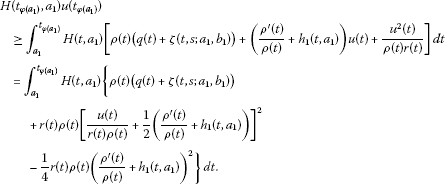It implies that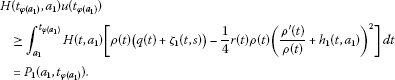(20)

On the other hand, multiplying both sides of (16) by $H\left({t}_{\phi \left({a}_{1}\right)+1},t\right)$, integrating it from ${t}_{\phi \left({a}_{1}\right)}$ to ${t}_{\phi \left({a}_{1}\right)+1}$, and similar to the above analysis, we can get

$-H\left({t}_{\phi \left({a}_{1}\right)+1},{t}_{\phi \left({a}_{1}\right)}\right)\frac{{d}_{\phi \left({a}_{1}\right)}}{{c}_{\phi \left({a}_{1}\right)}}u\left({t}_{\phi \left({a}_{1}\right)}\right)\ge {P}_{2}\left({t}_{\phi \left({a}_{1}\right)},{t}_{\phi \left({a}_{1}\right)+1}\right).$
(21)

Dividing (20) and (21) by $H\left({t}_{\phi \left({a}_{1}\right)},{a}_{1}\right)$ and $\frac{{d}_{\phi \left({a}_{1}\right)}}{{c}_{\phi \left({a}_{1}\right)}}H\left({t}_{\phi \left({a}_{1}\right)+1},{t}_{\phi \left({a}_{1}\right)}\right)$, respectively, and adding them, we have

$0\ge \frac{1}{H\left({t}_{\phi \left({a}_{1}\right)},{a}_{1}\right)}{P}_{1}\left({a}_{1},{t}_{\phi \left({a}_{1}\right)}\right)+\frac{{c}_{\phi \left({a}_{1}\right)}}{{d}_{\phi \left({a}_{1}\right)}}\frac{1}{H\left({t}_{\phi \left({a}_{1}\right)+1},{t}_{\phi \left({a}_{1}\right)}\right)}{P}_{2}\left({t}_{\phi \left({a}_{1}\right)},{t}_{\phi \left({a}_{1}\right)+1}\right).$

For the remaining intervals, similar to the analysis in Theorem 2.2 in , we have that for $t\in \left({a}_{1},{b}_{1}\right)$,

$\begin{array}{rcl}0& \ge & \frac{1}{H\left({t}_{\phi \left({a}_{1}\right)},{a}_{1}\right)}{P}_{1}\left({a}_{1},{t}_{\phi \left({a}_{1}\right)}\right)\\ +\sum _{n=1}^{n=\frac{{n}_{1}-1}{2}}\frac{1}{H\left({t}_{\phi \left({a}_{1}\right)+2n},{t}_{\phi \left({a}_{1}\right)+2n-1}\right)}{P}_{1}\left({t}_{\phi \left({a}_{1}\right)+2n-1},{t}_{\phi \left({a}_{1}\right)+2n}\right)\\ +\sum _{n=0}^{n=\frac{{n}_{1}-3}{2}}\frac{{c}_{\phi \left({a}_{1}\right)+2n}}{{d}_{\phi \left({a}_{1}\right)+2n}}\frac{1}{H\left({t}_{\phi \left({a}_{1}\right)+2n+1},{t}_{\phi \left({a}_{1}\right)+2n}\right)}{P}_{2}\left({t}_{\phi \left({a}_{1}\right)+2n},{t}_{\phi \left({a}_{1}\right)+2n+1}\right)\\ +\frac{{c}_{\phi \left({a}_{1}\right)+{n}_{1}-1}}{{d}_{\phi \left({a}_{1}\right)+{n}_{1}-1}}\frac{1}{H\left({b}_{1},{t}_{\phi \left({a}_{1}\right)+{n}_{1}-1}\right)}{P}_{2}\left({t}_{\phi \left({a}_{1}\right)+{n}_{1}-1},{b}_{1}\right).\end{array}$
(22)

It can be concluded similarly for the case (ii) that

$\begin{array}{rcl}0& \ge & \frac{1}{H\left({t}_{\phi \left({a}_{1}\right)},{a}_{1}\right)}{P}_{1}\left({a}_{1},{t}_{\phi \left({a}_{1}\right)}\right)\\ +\sum _{n=1}^{n=\frac{{n}_{1}-2}{2}}\frac{1}{H\left({t}_{\phi \left({a}_{1}\right)+2n},{t}_{\phi \left({a}_{1}\right)+2n-1}\right)}{P}_{1}\left({t}_{\phi \left({a}_{1}\right)+2n-1},{t}_{\phi \left({a}_{1}\right)+2n}\right)\\ +\sum _{n=0}^{n=\frac{{n}_{1}-2}{2}}\frac{{c}_{\phi \left({a}_{1}\right)+2n}}{{d}_{\phi \left({a}_{1}\right)+2n}}\frac{1}{H\left({t}_{\phi \left({a}_{1}\right)+2n+1},{t}_{\phi \left({a}_{1}\right)+2n}\right)}{P}_{2}\left({t}_{\phi \left({a}_{1}\right)+2n},{t}_{\phi \left({a}_{1}\right)+2n+1}\right)\\ +\frac{1}{H\left({\chi }_{1},{t}_{\phi \left({a}_{1}\right)+{n}_{1}-1}\right)}{P}_{1}\left({t}_{\phi \left({a}_{1}\right)+{n}_{1}-1},{\chi }_{1}\right)\\ +\frac{1}{H\left({b}_{1},{\chi }_{1}\right)}{P}_{2}\left({\chi }_{1},{b}_{1}\right),\phantom{\rule{1em}{0ex}}t\in \left({a}_{1},{b}_{1}\right).\end{array}$
(23)

We see that (22) and (23) contradict (18) and (19), respectively. The proof is complete. □

## 4 Examples

In this section, we give two examples to illustrate our main results. To simplify the computation, we focus our attention on the simple case $\xi \left(s\right)=s$ for Eq. (1).

Example 4.1 Consider the following impulsive differential equation:

$\left\{\begin{array}{c}{x}^{″}\left(t\right)+x\left(t\right)+\beta sint{\int }_{0}^{1}{|x\left(t-\frac{\pi }{4}\right)|}^{\frac{3\sqrt{s}}{2}}sgnx\left(t-\frac{\pi }{4}\right)\phantom{\rule{0.2em}{0ex}}ds=e\left(t\right),\phantom{\rule{1em}{0ex}}t\ne {t}_{k},\hfill \\ x\left({t}_{k}^{+}\right)={c}_{k}x\left({t}_{k}^{-}\right),\phantom{\rule{2em}{0ex}}{x}^{\prime }\left({t}_{k}^{+}\right)={d}_{k}{x}^{\prime }\left({t}_{k}^{-}\right),\hfill \end{array}$
(24)

where β is a positive constant, ${c}_{k}\ge 1$, ${d}_{k}>0$ are constants, $t\ge 0$, ${t}_{k}=2k\pi +\frac{\pi }{4}$ for $k=0,1,2,\dots$ , $r\left(t\right)=q\left(t\right)=1$, $p\left(t,s\right)=\beta sint$, $\tau \left(t,s\right)=t-\frac{\pi }{4}$, $\alpha \left(s\right)=\frac{3\sqrt{s}}{2}$ and $h=1$. For any $T\ge 0$, we choose k large enough such that $2k\pi \ge T$ and let ${a}_{1}=2k\pi$, ${a}_{2}={b}_{1}=2k\pi +\frac{\pi }{2}$ and ${b}_{2}=2k\pi +\pi$. Then we have $p\left(t,s\right)\ge 0$ for $t\in \left({a}_{1},{b}_{1}\right)\cup \left({a}_{2},{b}_{2}\right)$. Assume that $e\left(t\right)\in C\left[0,\mathrm{\infty }\right)$ is any function satisfying ${\left(-1\right)}^{j}e\left(t\right)\ge 0$ on $\left[{a}_{j},{b}_{j}\right]$ ($j=1,2$). Let $\eta \left(s\right)=1$. It is easy to verify that (10) and (11) are valid for $\delta =1$,

$R\left({\tau }_{{a}_{1}{b}_{1}},t\right)={\int }_{t-\frac{\pi }{4}}^{t}\frac{1}{r\left(s\right)}\phantom{\rule{0.2em}{0ex}}ds=\frac{\pi }{4},\phantom{\rule{1em}{0ex}}t\in \left(2k\pi ,2k\pi +\frac{\pi }{4}\right],$

and

$\begin{array}{rcl}R\left({\tau }_{{a}_{1}{b}_{1}},t\right)& =& {\int }_{2k\pi +\frac{\pi }{4}}^{t}\frac{ds}{r\left(s\right)}+\frac{1}{{d}_{k}}{\int }_{t-\frac{\pi }{4}}^{2k\pi +\frac{\pi }{4}}\frac{ds}{r\left(s\right)}\\ =& t-2k\pi -\frac{\pi }{4}+\frac{1}{{d}_{k}}\left(2k\pi +\frac{\pi }{2}-t\right)\\ =& \frac{\left({d}_{k}-1\right)\left(4t-8k\pi -\pi \right)+\pi }{4{d}_{k}},\phantom{\rule{1em}{0ex}}t\in \left(2k\pi +\frac{\pi }{4},2k\pi +\frac{\pi }{2}\right).\end{array}$

Since

$m\left(t,s;{a}_{1},{b}_{1}\right)={\left[exp\left({\int }_{t-\frac{\pi }{4}}^{t}\frac{1}{R\left({\tau }_{{a}_{1}{b}_{1}},u\right)}\phantom{\rule{0.2em}{0ex}}du\right)\right]}^{-1}\prod _{k=\phi \left(t-\frac{\pi }{4}\right)}^{\psi \left(t\right)}\frac{1}{{c}_{k}},$

we have

${m}_{1}\left(t,s;{a}_{1},{b}_{1}\right)=\left\{\begin{array}{cc}\frac{1}{e},\hfill & t\in \left(2k\pi ,2k\pi +\frac{\pi }{4}\right];\hfill \\ \frac{1}{{c}_{k}}{\left[\frac{\left({d}_{k}-1\right)\left(4t-8k\pi -\pi \right)+\pi }{\left({d}_{k}-1\right)\left(4t-8k\pi -2\pi \right)+\pi }\right]}^{\frac{{d}_{k}}{1-{d}_{k}}},\hfill & t\in \left(2k\pi +\frac{\pi }{4},2k\pi +\frac{\pi }{2}\right),\hfill \end{array}$

and

$\zeta \left(t,s;{a}_{1},{b}_{1}\right)=exp\left({\int }_{0}^{1}ln\left(\beta sint{\left[m\left(t,s;{a}_{1},{b}_{1}\right)\right]}^{\frac{3\sqrt{s}}{2}}\right)\phantom{\rule{0.2em}{0ex}}ds\right).$

Similarly, for $j=2$, i.e., $t\in \left(2k\pi +\frac{\pi }{2},2k\pi +\pi \right)$, we have $m\left(t,s;{a}_{2},{b}_{2}\right)=\frac{1}{e}$, and

$\begin{array}{rcl}\zeta \left(t,s;{a}_{2},{b}_{2}\right)& =& exp\left({\int }_{0}^{1}ln\left(\beta sint{\left[m\left(t,s;{a}_{2},{b}_{2}\right)\right]}^{\frac{3\sqrt{s}}{2}}\right)\phantom{\rule{0.2em}{0ex}}ds\right)\\ =& \frac{\beta sint}{e}.\end{array}$

Choose $\rho \left(t\right)=1$ and $\omega \left(t\right)=sin4t$. By Theorem 3.1, we know that Eq. (24) is oscillatory if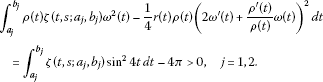Example 4.2 Consider the following equation:

$\left\{\begin{array}{c}{x}^{″}\left(t\right)+x\left(t\right)+{\int }_{0}^{1}{|x\left(t-1\right)|}^{2s}sgnx\left(t-1\right)\phantom{\rule{0.2em}{0ex}}ds=e\left(t\right),\phantom{\rule{1em}{0ex}}t\ne {t}_{k},\hfill \\ x\left({t}_{k}^{+}\right)={c}_{k}x\left({t}_{k}^{-}\right),\phantom{\rule{2em}{0ex}}{x}^{\prime }\left({t}_{k}^{+}\right)={d}_{k}{x}^{\prime }\left({t}_{k}^{-}\right),\hfill \end{array}$
(25)

where ${c}_{k}\ge 1$, ${d}_{k}>0$ are constants, $t\ge 0$, ${t}_{k}=4k-3$ ($k=1,2,\dots$), $r\left(t\right)=q\left(t\right)=p\left(t,s\right)=1$, $\tau \left(t,s\right)=t-1$, and $\alpha \left(s\right)=2s$. For any $T>0$, we choose k large enough such that $4\left(k-1\right)\ge T$ and let ${a}_{1}=4\left(k-1\right)$, ${a}_{2}={b}_{1}=4k-2$ and ${b}_{2}=4k$. Then we have $p\left(t,s\right)\ge 0$ for $t\in \left({a}_{1},{b}_{1}\right)\cup \left({a}_{2},{b}_{2}\right)$. Assume that $e\left(t\right)\in C\left[0,\mathrm{\infty }\right)$ is any function satisfying ${\left(-1\right)}^{j}e\left(t\right)\ge 0$ on $\left[{a}_{j},{b}_{j}\right]$ ($j=1,2$). For any $\delta \in \left(1/2,1\right]$, set

$\eta \left(s\right)=\frac{\delta }{2\delta -1}{s}^{\left(2-2\delta \right)/\left(2\delta -1\right)}.$

It is easy to verify that (10) and (11) are valid. Let $\rho \left(t\right)=1$ and $H\left(t,s\right)={\left(t-s\right)}^{2}$. We have that ${h}_{1}\left(t,s\right)={h}_{2}\left(t,s\right)\equiv 1$. For $j=1$, we have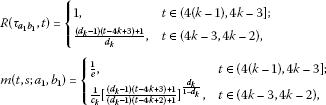and

$\zeta \left(t,s;{a}_{1},{b}_{1}\right)={\left[\frac{|e\left(t\right)|}{1-\delta }\right]}^{1-\delta }exp\left({\int }_{0}^{1}\eta \left(s\right)ln\frac{{\left[m\left(t,s;{a}_{1},{b}_{1}\right)\right]}^{2s}}{\eta \left(s\right)}\phantom{\rule{0.2em}{0ex}}ds\right).$

For $t\in \left(4\left(k-1\right),4k-2\right)$, we obtain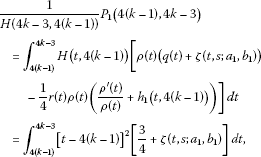and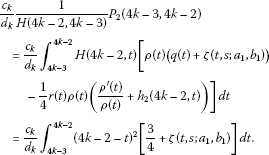Similarly, for $t\in \left(4k-2,4k\right)$, we have that $m\left(t,s;{a}_{2},{b}_{2}\right)=\frac{1}{e}$, and

$\begin{array}{rcl}\zeta \left(t,s;{a}_{2},{b}_{2}\right)& =& {\left[\frac{|e\left(t\right)|}{1-\delta }\right]}^{1-\delta }exp\left({\int }_{0}^{1}\eta \left(s\right)ln\frac{{\left[m\left(t,s;{a}_{2},{b}_{2}\right)\right]}^{2s}}{\eta \left(s\right)}\phantom{\rule{0.2em}{0ex}}ds\right)\\ =& {\left[\frac{|e\left(t\right)|}{1-\delta }\right]}^{1-\delta }exp\left(-{\int }_{0}^{1}\eta \left(s\right)\left(2s+ln\eta \left(s\right)\right)\phantom{\rule{0.2em}{0ex}}ds\right).\end{array}$

We choose $\chi =4k-1$, so we have

$\frac{1}{H\left(\chi ,4k-2\right)}{P}_{1}\left(4k-2,\chi \right)={\int }_{4k-2}^{4k-1}{\left[t-\left(4k-2\right)\right]}^{2}\left[1+\zeta \left(t,s;{a}_{2},{b}_{2}\right)-\frac{1}{4}\right]\phantom{\rule{0.2em}{0ex}}dt,$

and

$\frac{1}{H\left(4k,\chi \right)}{P}_{2}\left(\chi ,4k\right)={\int }_{4k-1}^{4k}{\left(4k-t\right)}^{2}\left[\frac{3}{4}+\zeta \left(t,s;{a}_{2},{b}_{2}\right)\right]\phantom{\rule{0.2em}{0ex}}dt.$

From Theorem 3.2 we know that Eq. (25) is oscillatory if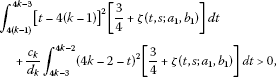and

${\int }_{4k-2}^{4k-1}{\left[t-\left(4k-2\right)\right]}^{2}\left[\frac{3}{4}+\zeta \left(t,s;{a}_{2},{b}_{2}\right)\right]\phantom{\rule{0.2em}{0ex}}dt+{\int }_{4k-1}^{4k}{\left(4k-t\right)}^{2}\left[\frac{3}{4}+\zeta \left(t,s;{a}_{2},{b}_{2}\right)\right]\phantom{\rule{0.2em}{0ex}}dt>0.$

## References

1. Ballinger G, Liu X: Permanence of population growth models with impulsive effects. Math. Comput. Model. 1997, 26: 59–72.

2. Lu Z, Chi X, Chen L: Impulsive control strategies in biological control of pesticide. Theor. Popul. Biol. 2003, 64: 39–47. 10.1016/S0040-5809(03)00048-0

3. Sun J, Qiao F, Wu Q: Impulsive control of a financial model. Phys. Lett. A 2005, 335: 282–288. 10.1016/j.physleta.2004.12.030

4. Tang S, Chen L: Global attractivity in a food-limited population model with impulsive effect. J. Math. Anal. Appl. 2004, 292: 211–221. 10.1016/j.jmaa.2003.11.061

5. Tang S, Xiao Y, Clancy D: New modelling approach concerning integrated disease control and cost-effectivity. Nonlinear Anal. 2005, 63: 439–471. 10.1016/j.na.2005.05.029

6. Zhang Y, Xiu Z, Chen L: Dynamics complexity of a two-prey one-predator system with impulsive effect. Chaos Solitons Fractals 2005, 26: 131–139. 10.1016/j.chaos.2004.12.037

7. Lakshmikantham V, Bainov DD, Simeonov PS Series in Modern Applied Mathematics 6. In Theory of Impulsive Differential Equations. World Scientific, Singapore; 1989.

8. Benchohra M, Henderson J, Ntouyas S Contemporary Mathematics and Its Applications 2. In Impulsive Differential Equations and Inclusions. Hindawi Publishing Corporation, New York; 2006.

9. Bainov D, Covachev V: Impulsive Differential Equations with a Small Parameter. World Scientific, Singapore; 1995.

10. Agarwa RP, Karakoç F: Survey on oscillation of impulsive delay differential equations. Comput. Math. Appl. 2010, 60: 1648–1685. 10.1016/j.camwa.2010.06.047

11. Özbekler A, Zafer A: Forced oscillation of super-half-linear impulsive differential equations. Comput. Math. Appl. 2007, 54: 785–792. 10.1016/j.camwa.2007.03.003

12. Özbekler A, Zafer A: Interval criteria for the forced oscillation of super-half-linear differential equations under impulse effects. Math. Comput. Model. 2009, 50: 59–65. 10.1016/j.mcm.2008.10.020

13. Luo Z, Shen J: Oscillation of second order linear differential equations with impulses. Appl. Math. Lett. 2007, 20: 75–81. 10.1016/j.aml.2006.01.019

14. Chen YS, Feng WZ: Oscillation of second order nonlinear ODE with impulses. J. Math. Anal. Appl. 1997, 210: 150–169. 10.1006/jmaa.1997.5378

15. Liu XX, Xu ZT: Oscillation of a forced super-linear second order differential equation with impulses. Comput. Math. Appl. 2007, 53: 1740–1749. 10.1016/j.camwa.2006.08.040

16. Liu XX, Xu ZT: Oscillation criteria for a forced mixed typed Emden-Fowler equation with impulses. Comput. Math. Appl. 2009, 215: 283–291. 10.1016/j.amc.2009.04.072

17. Muthulakshmi V, Thandapani E: Interval criteria for oscillation of second-order impulsive differential equation with mixed nonlinearities. Electron. J. Differ. Equ. 2011, 2011(40):1–14.

18. Özbekler A, Zafer A: Oscillation of solutions of second order mixed nonlinear differential equations under impulsive perturbations. Comput. Math. Appl. 2011, 61: 933–940. 10.1016/j.camwa.2010.12.041

19. Guo Z, Zhou X, Ge W: Interval oscillation criteria for second-order forced impulsive differential equations with mixed nonlinearities. J. Math. Anal. Appl. 2011, 381: 187–201. 10.1016/j.jmaa.2011.02.073

20. Zafer A: Oscillation of second-order sublinear impulsive differential equations. Abstr. Appl. Anal. 2011., 2011: Article ID 458275

21. Sun YG, Kong QK: Interval criteria for forced oscillation with nonlinearities given by Riemann-Stieltjes integrals. Comput. Math. Appl. 2011, 62: 243–252. 10.1016/j.camwa.2011.04.072

22. Sun YG, Wong JSW: Oscillation criteria for second order forced ordinary differential equations with mixed nonlinearities. J. Math. Anal. Appl. 2007, 334: 549–560. 10.1016/j.jmaa.2006.07.109

23. Sun YG, Meng FW: Oscillation of second-order delay differential equations with mixed nonlinearities. Comput. Math. Appl. 2009, 207: 135–139. 10.1016/j.amc.2008.10.016

24. Liu Z, Sun YG: Interval criteria for oscillation of a forced impulsive differential equation with Riemann-Stieltjes integral. Comput. Math. Appl. 2012, 63: 1577–1586. 10.1016/j.camwa.2012.03.010

25. Kong Q: Interval criteria for oscillation of second order linear ordinary differential equations. J. Math. Anal. Appl. 1999, 229: 258–270. 10.1006/jmaa.1998.6159

26. El-Sayed MA: An oscillation criterion for a forced second order linear differential equation. Proc. Am. Math. Soc. 1993, 118: 813–817.

27. Yang X: Oscillation criteria for nonlinear differential equations with damping. Comput. Math. Appl. 2003, 136: 549–557. 10.1016/S0096-3003(02)00079-6

28. Hassan TS, Kong Q: Interval criteria for forced oscillation of differential equations with p -Laplacian and nonlinearities given by Riemann-Stieltjes integrals. J. Korean Math. Soc. 2012, 49: 1017–1030. 10.4134/JKMS.2012.49.5.1017

29. Erbe L, Hassan TS, Peterson A: Oscillation criteria for nonlinear damped dynamic equations on time scales. Comput. Math. Appl. 2008, 203: 343–357. 10.1016/j.amc.2008.04.038

## Acknowledgements

This work was supported by the National Natural Science Foundation of China (Grant No. 61174217) and the Natural Science Foundation of Shandong Province (Grant Nos. ZR2010AL002 and JQ201119).

## Author information

Authors

### Corresponding author

Correspondence to Yuangong Sun.

### Competing interests

The authors declare that they have no competing interests.

### Authors’ contributions

All authors carried out the proof. All authors conceived of the study and participated in its design and coordination. All authors read and approved the final manuscript.

## Rights and permissions

Open Access This article is distributed under the terms of the Creative Commons Attribution 2.0 International License (https://creativecommons.org/licenses/by/2.0), which permits unrestricted use, distribution, and reproduction in any medium, provided the original work is properly cited.

Reprints and Permissions

Liu, Z., Sun, Y. Oscillation of impulsive functional differential equations with oscillatory potentials and Riemann-Stieltjes integrals. Adv Differ Equ 2012, 175 (2012). https://doi.org/10.1186/1687-1847-2012-175

• Accepted:

• Published:

• DOI: https://doi.org/10.1186/1687-1847-2012-175

### Keywords

• oscillation
• impulse
• delay
• Riemann-Stieltjes integral